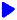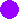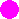Noetic Learning Math Worksheet Creator

Worksheets for Pre-Algebra3Back to Worksheet Creator HomeSolve one-step equations   free sample

equations with numbers up to

include negative numbers in questions

Number of questions:   questions per row: Generate!Solve two-step equations     free sample

with numbers up to

include negative numbers in questions

Number of questions: questions per row:  Generate!